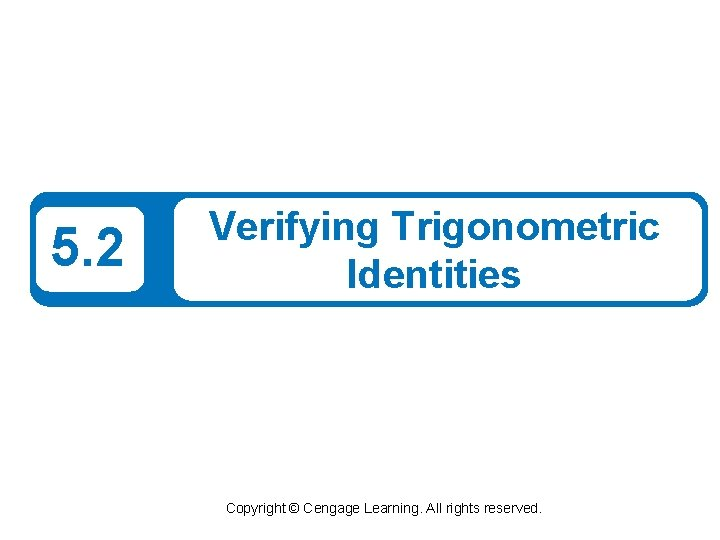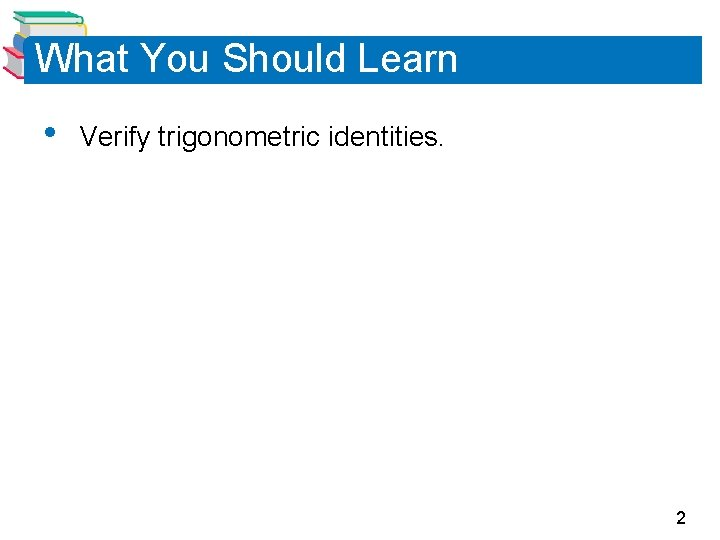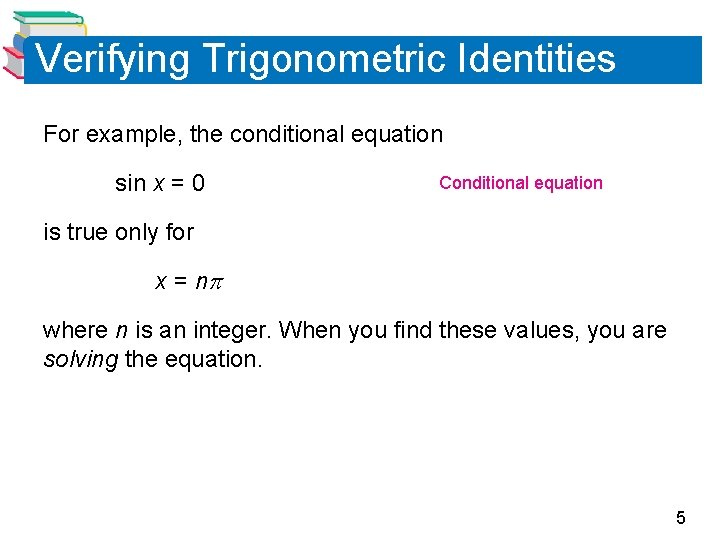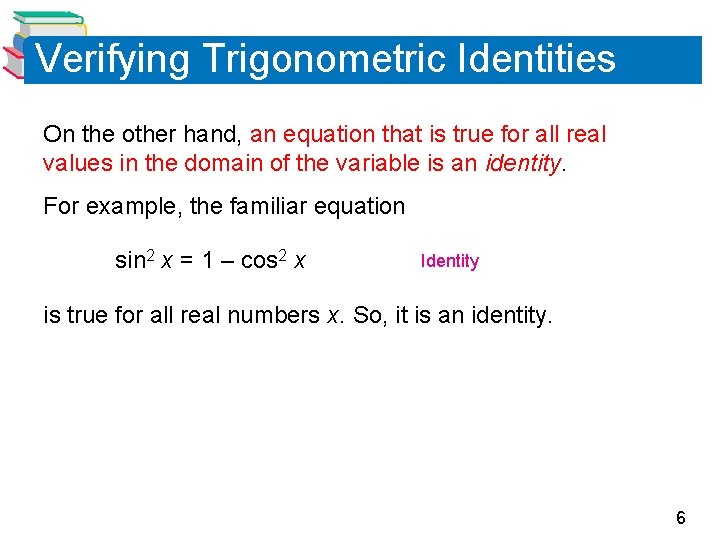# 5 2 Verifying Trigonometric Identities Copyright Cengage Learning

• Slides: 9What You Should Learn • Verify trigonometric identities. 2Verifying Trigonometric Identities 3Verifying Trigonometric Identities In this section, you will study techniques for verifying trigonometric identities. In the next section, you will study techniques for solving trigonometric equations. The key to both verifying identities and solving equations is your ability to use the fundamental identities and the rules of algebra to rewrite trigonometric expressions. Remember that a conditional equation is an equation that is true for only some of the values in its domain. 4Verifying Trigonometric Identities For example, the conditional equation sin x = 0 Conditional equation is true only for x = n where n is an integer. When you find these values, you are solving the equation. 5Verifying Trigonometric Identities On the other hand, an equation that is true for all real values in the domain of the variable is an identity. For example, the familiar equation sin 2 x = 1 – cos 2 x Identity is true for all real numbers x. So, it is an identity. 6Verifying Trigonometric Identities 7Example 1 – Verifying a Trigonometric Identity Verify the identity. Solution: Because the left side is more complicated, start with it. Pythagorean identity 8Example 1 – Solution cont’d Simplify. = tan 2 (cos 2 ) Reciprocal identity Quotient identity = sin 2 Simplify. 9